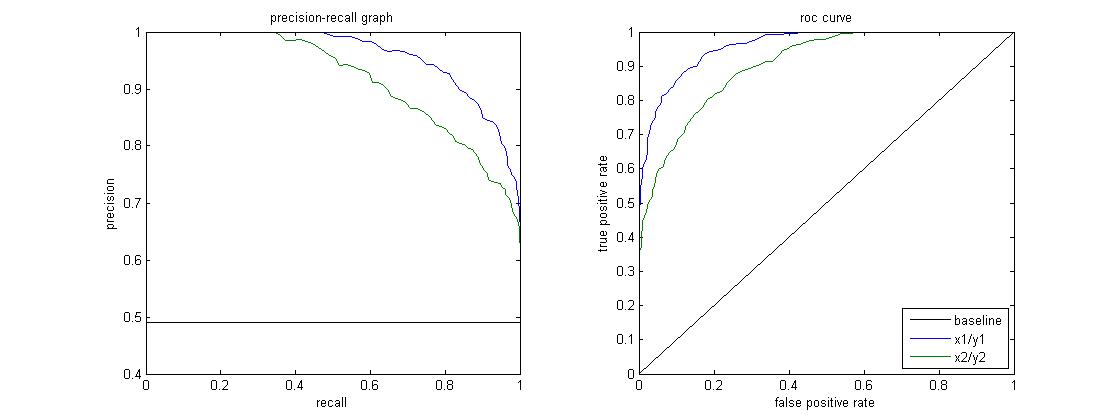Open In App
Related Articles
• Write an Interview Experience
• Machine Learning Algorithms
• Precision-Recall Curve | ML

# Precision-Recall Curve | ML

There are numerous ways to evaluate the performance of a classifier. In this article, we introduce the Precision-Recall Curve and further examine the difference between two popular performance reporting methods: Precision-Recall (PR) Curve and Receiver Operating Characteristic (ROC) Curve. ROC Curve is already discussed in the article. Let us briefly understand what is a Precision-Recall curve.

## Precision-Recall (PR) Curve –

Precision is the proportion of correct positive classifications (true positive) divided by the total number of predicted positive classifications that were made (true positive + false positive). Recall is the proportion correct positive classifications (true positive) divided by the total number of the truly positive classifications (true positive + false negative).

A PR curve is simply a graph with Precision values on the y-axis and Recall values on the x-axis. In other words, the PR curve contains TP/(TP+FP) on the y-axis and TP/(TP+FN) on the x-axis.

• It is important to note that Precision is also called the Positive Predictive Value (PPV).
• Recall is also called Sensitivity, Hit Rate or True Positive Rate (TPR).

The figure below shows a juxtaposition of sample PR and ROC curves.## Interpreting a PR Curve –

It is desired that the algorithm should have both high precision, and high recall. However, most machine learning algorithms often involve a trade-off between the two. A good PR curve has greater AUC (area under curve). In the figure above, the classifier corresponding to the blue line has better performance than the classifier corresponding to the green line. It is important to note that the classifier that has a higher AUC on the ROC curve will always have a higher AUC on the PR curve as well. Consider an algorithm that classifies whether or not a document belongs to the category “Sports” news. Assume there are 12 documents, with the following ground truth (actual) and classifier output class labels.

Now, let us find TP, TN, FP and FN values.

TP = The document was classified as “Sports” and was actually “Sports”. D1, D2, D10, and D11 correspond to TP. TN = The document was classified as “Not sports” and was actually “Not sports”. D5, D8, and D9 correspond to TN. FP = The document was classified as “Sports” but was actually “Not sports”. D3 and D7 correspond to FP. FN = The document was classified as “Not sports” but was actually “Sports”. D4, D6, and D12 correspond to FN. So, TP = 4, TN = 3, FP = 2 and FN = 3. Finally, precision = TP/(TP+FP) = 4/6 = 2/3 and recall = TP/(TP+FN) = 4/7. This means when the precision is 2/3, the recall is 4/7.

By setting different thresholds, we get multiple such precision, recall pairs. By plotting multiple such P-R pairs with either value ranging from 0 to 1, we get a PR curve.

## Need for a PR curve when the ROC curve exists ?

PR curve is particularly useful in reporting Information Retrieval results.
Information Retrieval involves searching a pool of documents to find ones which are relevant to a particular user query. For instance, assume that the user enters a search query “Pink Elephants”. The search engine skims through millions of documents (using some optimized algorithms) to retrieve a handful of relevant documents. Hence, we can safely assume that the no. of relevant documents will be very less compared to the no. of non-relevant documents. In this scenario, TP = No. of retrieved documents that are actually relevant (good results). FP = No. of retrieved documents that are actually non-relevant (bogus search results). TN = No. of non-retrieved documents that are actually non-relevant. FN = No. of non-retrieved documents that are actually relevant (good documents we missed). ROC curve is a plot containing Recall = TPR = TP/(TP+FN) on the x-axis and FPR = FP/(FP+TN) on the y-axis. Since the no. of true negatives, i.e. non-retrieved documents that are actually non-relevant, is such a huge number, the FPR becomes insignificantly small. Further, FPR does not really help us evaluate a retrieval system well because we want to focus more on the retrieved documents, and not the non-retrieved ones. PR curve helps solve this issue. PR curve has the Recall value (TPR) on the x-axis, and precision = TP/(TP+FP) on the y-axis. Precision helps highlight how relevant the retrieved results are, which is more important while judging an IR system. Hence, a PR curve is often more common around problems involving information retrieval.